# Electron Geometry VS Molecular Geometry

Electron geometry and molecular geometry are the arrangements of electrons or atoms in three-dimensional space around a central atom. This gives a molecule a specific shape and bond angles.

Electron geometry helps us in determining the arrangement of various electron groups. Molecular geometry, on the other hand, helps us in determining the entire atom and its configuration. It is the three-dimensional arrangement of all the atoms in a given molecule.

## Difference Between Electron Geometry and Molecular Geometry

 Molecular Geometry Electron Geometry The arrangement of atoms in a molecule, usually relative to a single central atom, is known as molecular geometry. The arrangement of electron pairs around a central atom is known as electron geometry. It excludes lone pairs from determining a molecule’s shape, though repulsion from lone pair(s) is taken into account only in bond angles. In determining the shape, it takes into account the presence of both bond pair(s) and lone pair(s) of electrons. Number of bonding pairs are only calculated. Number of total electron pairs are calculated.

Examples:

• In a water molecule, H2O two of the central oxygen atom’s valence electrons form two bond pairs with the hydrogen atoms, while the remaining four electrons form two lone pairs. Therefore, the molecular geometry of water is bent and the electron geometry of water is tetrahedral.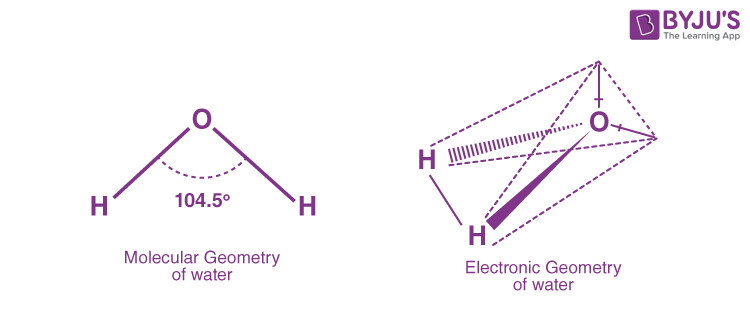• Ammonia, NH3, is another example with different molecular and electron geometries. The central atom nitrogen has three bond pairs and a lone pair of electrons with five valence electrons. It has trigonal pyramidal molecular geometry and tetrahedral electron geometry.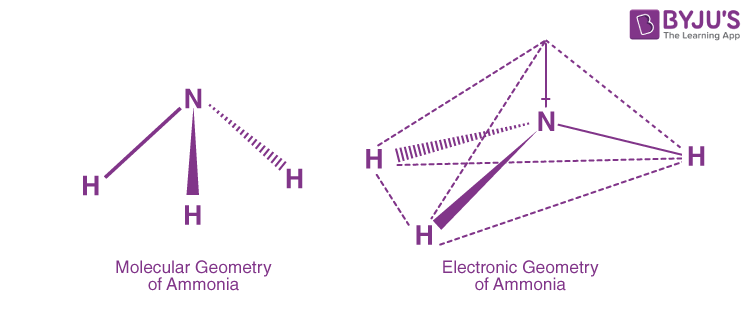Read more:Molecular Geometry and Electron Geometry

The chart below depicts some molecule geometries based on the number of electron pairs present.

 Number of electron pairs Number of bonding electron pairs Number of lone electron pairs Type Electron geometry Shape Molecular geometry Shape 2 2 0 AB2 Linear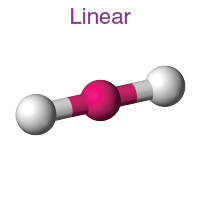Linear3 3 0 AB3 Trigonal planar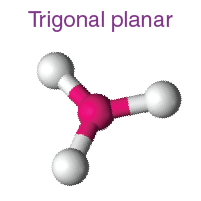Trigonal planar3 2 1 AB3 Trigonal planarBent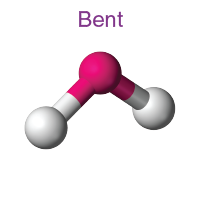4 4 0 AB4 Tetrahedral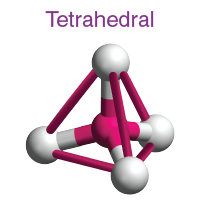Tetrahedral4 3 1 AB4 TetrahedralTrigonal pyramid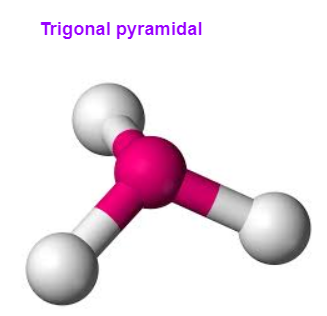4 2 2 AB4 TetrahedralBent5 5 0 AB5 Trigonal bipyramidal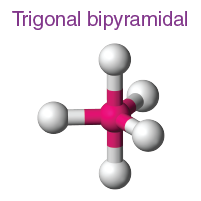Trigonal bipyramidal5 4 1 AB5 Trigonal bipyramidalSeesaw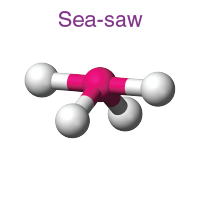5 3 2 AB5 Trigonal bipyramidalT-shaped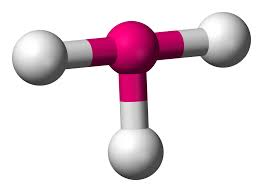5 2 3 AB5 Trigonal bipyramidalLinear6 6 0 AB6 Octahedral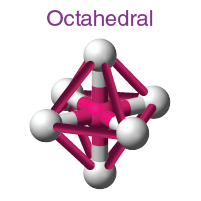Octahedral6 5 1 AB6 OctahedralSquare pyramidal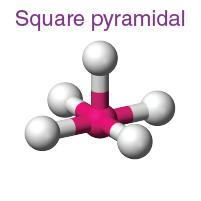6 4 2 AB6 OctahedralSquare planar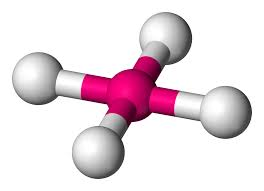6 2 4 AB6 OctahedralLinear## Determination of Electron Geometry

The steps taken in making the determination of electron geometry are as follows.

1. Predict the molecule’s central atom. It should be the atom with the highest electronegative potential.
2. The number of valence electrons in the central atom must be determined.
3. Calculate the total number of electrons donated by other atoms.
4. Determine the total number of electrons surrounding the central atom.
5. Subtract that number from two. This number represents the number of electron groups present.
6. Subtract the steric number from the number of single bonds present around the central atom. This number represents the number of lone electron pairs in the molecule.
7. Determine the geometry of the electron.

## Determination of Molecular Geometry

Lone pair electrons are not considered in this molecular geometry. Furthermore, double and triple bonds are regarded as single bonds. Since lone electron pairs require more space than bonding electron pairs, the geometries are determined by this fact.

Geometrical parameters such as bond lengths, bond angles, and torsional angles help determine the position of atoms as well as the general shape of a molecule.

It has an effect on the properties of a substance, such as its reactivity, colour, polarity, magnetism, biological activity, and phase of matter.

### AXE Method

The AXE method is a useful tabular idea for representing molecular geometries. The central atom is represented by A, each X by an atom bonded to A (or bond pair), and each E by a lone pair on the central atom.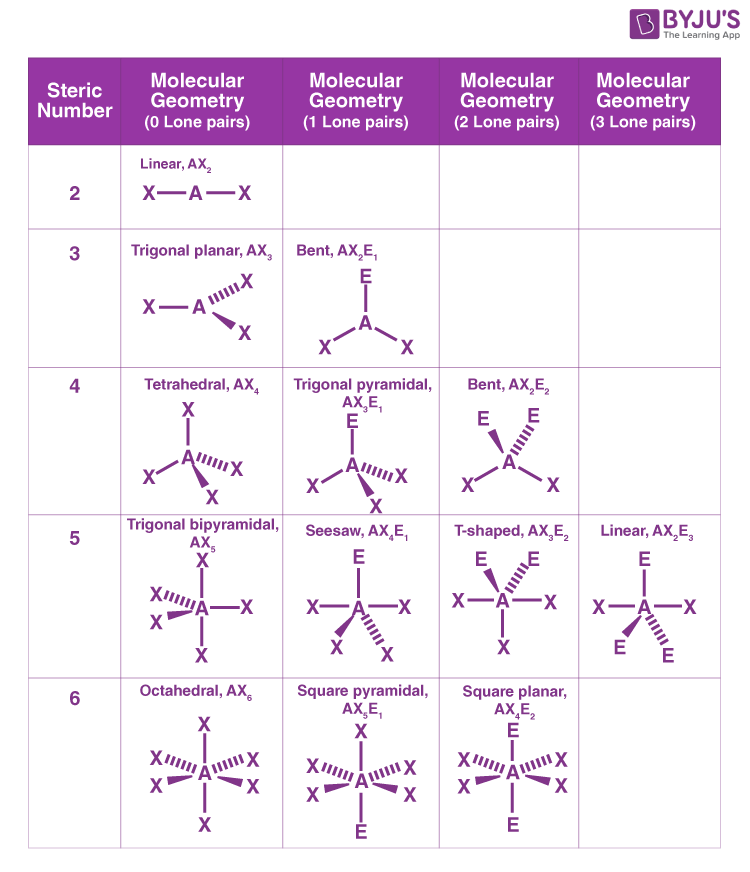## Frequently Asked Questions – FAQs

Q1

### Under what conditions are electro =n and molecular geometries not the same?

The arrangement of electron groups is referred to as electron geometry. The geometrical arrangements of the atoms are referred to as molecular geometry. The molecular geometry differs from the electron geometry when a molecule contains one or more lone pairs.

Q2

### Can molecular and electron geometry be the same?

Yes, when there are no lone pairs around the central atom in a molecule, the electron and molecular geometry are the same.

Q3

### What happens when atoms are replaced with lone pairs?

Due to the repulsion of lone pairs, the overall molecular shape (the molecule geometry) changes, but the electron geometry remains constant.

Q4

### What effect do bonds have on molecular shape?

The repulsion between electron pairs causes them to move as far away from each other as possible when a bond forms. This results in a molecule with a distinct geometrical pattern.

Q5

### Give an example of a compound with different electron and molecular geometry.

The central nitrogen atom in the NCl3 molecule has five valence electrons, 3 of which form bond pairs with chlorine atoms. As a result, NCl3 molecular geometry is trigonal pyramidal, and its electron geometry is tetrahedral.